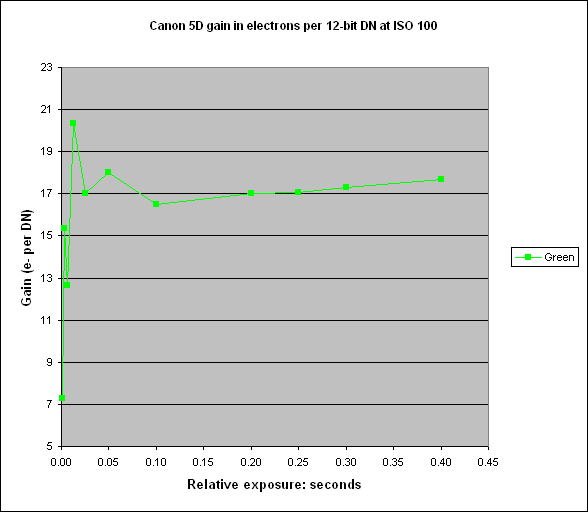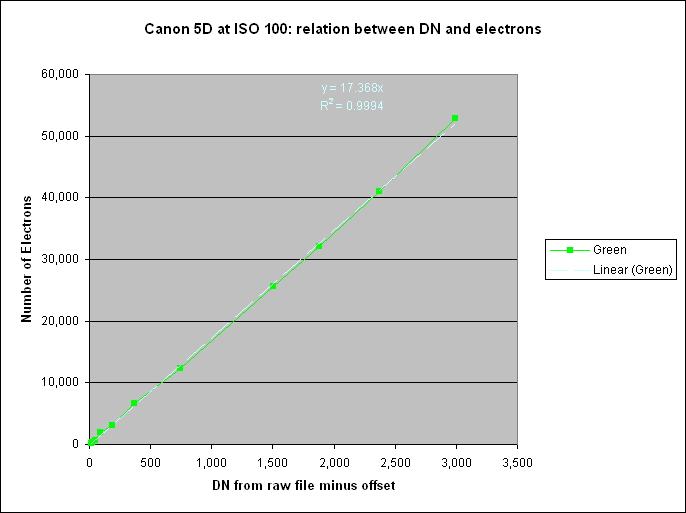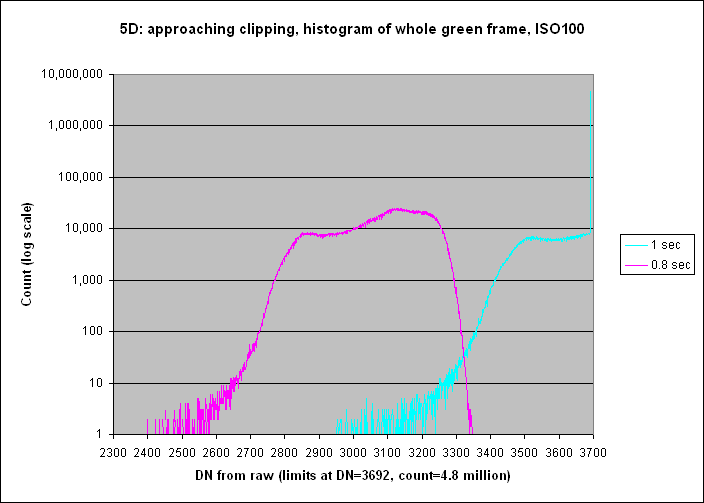Up to Canon 5D index

# Canon 5D gain and full well capacity at ISO 100

See here.

## Results

The table below shows statistics of a 200 x 200 pixel area in the centre of each image for the green component after the two frames have been subtracted.

The top six exposures are clipped by firmware at 3692, and this is the reason why their standard deviation is zero.

The number of electrons is calculated by squaring the signal-to-noise ratio, and the latter is calculated after quadratically subtracting the read noise from the measured noise.

The bottom four exposures are mainly just read noise, giving an inaccurate estimate for the number of electrons.

The gain is calculated by dividing the number of electrons by the Mean DN. It is plotted below:Ideally this should be a straight, horizontal line because the gain is, ex hypothesi, independent of exposure. It should vary only as a function of ISO setting.

The gain goes wild at low exposures, and would be wilder still had I not suppressed the points close to the origin. This is ok: it becomes increasingly difficult to measure the gain accurately as exposure reduces.

The gain is about 17 electrons per DN.

A better estimate of the gain can be got from plotting the number of electrons against DN:The white line shows the result of a linear regression on the eleven points where DN (minus offset) is between 12 and 2994. This region excludes the inaccurate points at the low end. The result, with intercept constrained to be zero, is:

Number of electrons = 17.368 * DN, R² = 0.9994

Hence I measure the gain of the Canon EOS 5D as 17.4 electrons per DN, in the green component of the Bayer array, when written to 12-bit CR2 files.

Since the 5D firmware clips all three colours at DN=3692, no measurement of the full well capacity is possible.

All we can say is that the content of the green wells of the Canon EOS 5D at the clipping point is (3692-128) * 17.368 = 61,900 electrons.

This graph shows histograms of the whole green component for two exposures, illustrating how clipping occurs:It isn't possible to deduce from this how far below saturation the clipping level is set, but one would assume that Canon would set the level not far below saturation otherwise it would artificially limit the dynamic range to no obvious purpose - unless of course there were a commercial purpose in giving the 5D a lower dynamic range than, say, the 1DsII.

Peter Facey, Winchester, England
20080130 originated